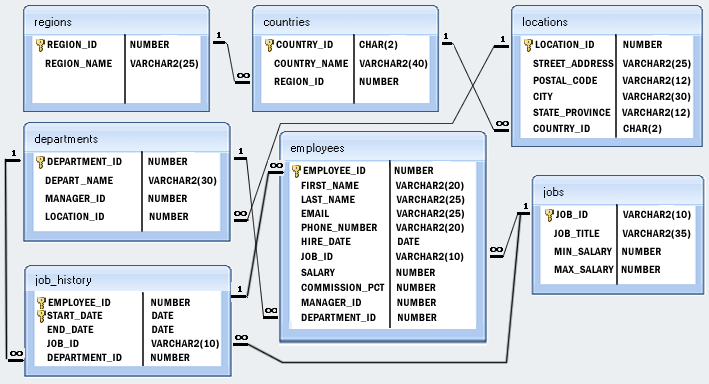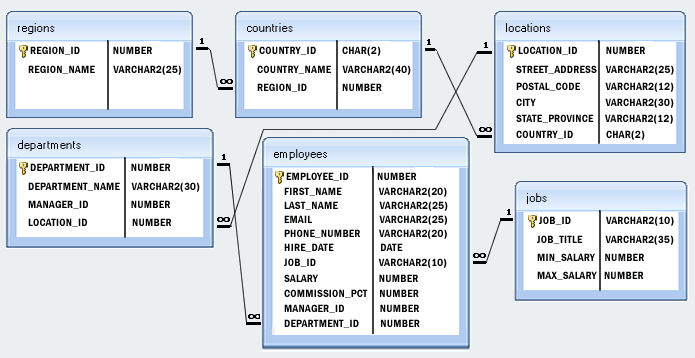﻿ SQLite Exercise: Calculate 171*214+625 - w3resource# SQLite Exercise: Calculate 171*214+625

Write a query to calculate 171*214+625.

SQLite Code :

``````SELECT 171*214+625 Result;
``````

Output:

```Result
----------------
37219
```

## Model DatabaseStructure of 'hr' database :Improve this sample solution and post your code through Disqus.

What is the difficulty level of this exercise?

﻿